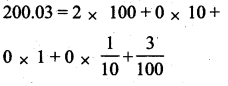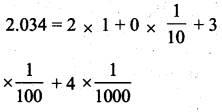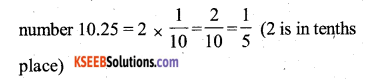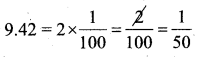# KSEEB Solutions for Class 7 Maths Chapter 2 Fractions and Decimals Ex 2.5

Students can Download Chapter 2 Fractions and Decimals Ex 2.5, Question and Answers, Notes Pdf, KSEEB Solutions for Class 7 Maths, Karnataka State Board Solutions help you to revise complete Syllabus and score more marks in your examinations.

## Karnataka State Syllabus Class 7 Maths Chapter 2 Fractions and Decimals Ex 2.5

Question 1.
Which is greater ?
i) 0.5 or 0.05
Solution:
0.5 > 0.05 (∵ 0.5 = 0.50)

ii) 0.7 or 0.5
Solution:
0.7 > 0.5

iii) 7 or 0.7
Solution:
7 > 0.7 (∵ 7 = 7.0)iv) 1.37 or 1.49
Solution:
1.49 > 1.37

v) 2.03 or 2.30
Solution:
2.30 > 2.03

vi) 0.8 or 0.88
Solution:
0.88 > 0.8 (∵ 0.8 = 80)

1 1/2 as a decimal is equal to 1.5.

Question 2.
Express as rupees using decimals :
i) 7 paise
Solution:
7 paise = Rs. 0.07

ii) 7 rupees 7 paise
Solution:
7 rupees 7 paise Rs. 7.07

iii) 77 rupees 77paise
Solution:
77 rupees 77 paise = ₹ 77.77iv) 50 paise
Solution:
50 paise = 0.50

v) 235 paise
Solution:
235 paise = 2.35

Question 3.
i) Express 5cm in metre and kilometre
Solution:
5 cm = 0.05m = 0.00005km.

ii) Express 35mm in cm, m and km
Solution:
35mm = 3.5cm = 0.000035km

Question 4.
Express in kg :
i) 200g
Solution:
200g = 0.200kg = 0.2 kg

ii) 3470g
Solution:
3470g = 3.470kg

iii) 4 kg 8 g
Solution:
4kg 8g = 4.008 kg

Question 5.
Write the following decimal numbers in the expanded form :
i) 20.03
Solution:ii) 2.03
Solution:iii) 200.03
Solution:iv) 2.034
Solution:Question 6.
Write the place value of 2 in the following decimal numbers :

i) 2.56
Solution:
2.56 place value of 2 in the decimal number 2.56 = 2 ones place)

ii) 21.37
Solution:
21.37 place value of 2 in the decimal number 21.37 = 2 × 10 = 20. (2 is in tens place)

iii) 10.25
Solution:
10.25 = palce value of 2 in the decimaliv) 9.42
Solution:
9.42 = place value of 2 in the decimal number(2 is in hundredths place)v) 63.352
Solution:
63.352 = place value of 2 in the decimal numberQuestion 7.
Dinesh went from place A to place B and from 12.7 km from C. Ayub went from place A to place D and from there to place C. D is 9.3 km from A and C is 11.8 km from D. Who travelles more and by how much ?
Solution:
Distance travelled from A to B = 7.5kms
Distance travelled from B to C = 12.7kms
Total distance travelled from A to B & B to C = 20.2 kms.
Ayub
Distance travelled from A to D = 9.3 kms
Distance travelled from D to C = 11.8 kms
Total distance travelled from A to D & D to C = 21.1 kms
∴ Ayub travelled more distance than Dinesh by = 21.1 – 20.2 = 0.9 kms.Question 8.
Shyama bought 5 kg 300g apples and 3 kg 250 g mangoes. Sarala bought 4 kg of 800 g oranges and 4 kg 150g bananas. Who bought more fruits?
Solution:
Shyama:
Weight of Apples bought = 5.300kgs
Weight of Mangoes bought = 3.250kgs
Total weight of fruits = 8.550kgs

Sarala:
Weight of oranges bought = 4.800kgs
Weight of Bananas bought = 4.150kgs
Total weight of fruits = 8.950kgs
8.950 >8.550
∴ Sarala bought more fruits than Shyama.Question 9.
How much less is 28 km than 42.6 km?
Solution:∴ 28 km is less than 42.6 km by 14.6 kms.

error: Content is protected !!